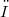﻿

### Su alcune proprietà ricorsive legate al concetto di densità

#### Abstract

In this paper we use the concept of density as a measure for subsets of$ω$, and we study the relations between density and recursive properties of subsets of$ω$. In § I we show that the set of those real numbers which express the density of a recursive set coincides with the set of weakly recursive real numbers (between 0 and 1, of course); in § II we investigate those sets whose density is recursively constant, i.e. those sets which have the same density of any recursive image of themselves; in § III we introduce the concept of pseudo-immune set and in § IV we analize the relations between our notion of density and the concept "at least dense as".

DOI Code: 10.1285/i15900932v1n1p137

Full Text: PDF